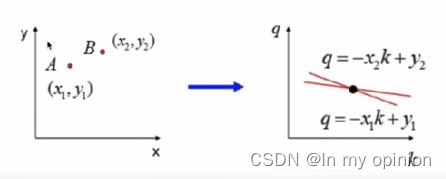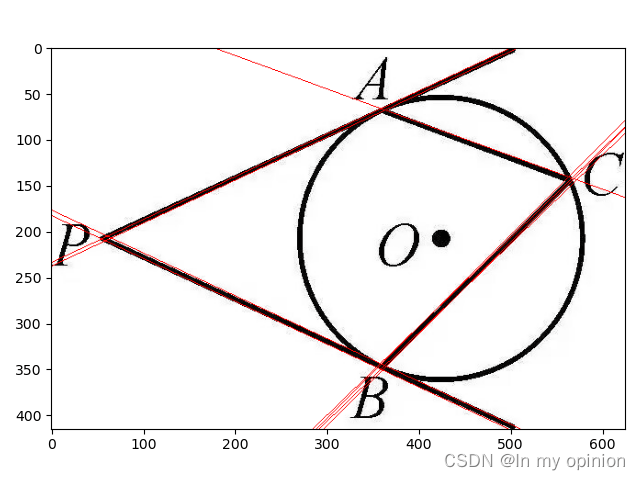1.定义：

2.实现：

1.原理：

2.实现：

三、霍夫圆检测

1.描述：

2.用法：

# 一、模板匹配

### 2.实现：

result=cv.matchTemplate( img,template,method)

1>template :模板
2>method: 实现模板匹配的算法，主要有:
1.平方差匹配(CV_TM_SQDIFF):利用模板与图像之间的平方差进行匹配，最好的匹配是0,匹配越差，匹配的值越大。
2.相关匹配(CV_TM_CCORR):利用模板与图像间的乘法进行匹配，数值越大表示匹配程度较高，越小表示匹配效果差。
3.利用相关系数匹配(CV_TM_CCOEFF):利用模板与图像间的相关系数匹配，1表示完美的匹配，-1表示最差的匹配。

``````import numpy as np
import matplotlib.pyplot as plt
import cv2 as cv
#plt.imshow(img[:,:,::-1])
#plt.show()
h,w=template.shape[:2]
result=cv.matchTemplate(img,template,cv.TM_CCORR)
min_val,max_val,min_loc,max_loc=cv.minMaxLoc(result)
top_left=max_loc
bottom_right=(top_left+w,top_left+h)
cv.rectangle(img,top_left,bottom_right,(0,255,0),2)#设置颜色与宽度
plt.imshow(img[:,:,::-1])
plt.show()``````# 二、霍夫线检测

### 1.原理：### 2.实现：

cv.HoughLines(img,rho,theta,threshold)

img:检测的图像，要求是二值化的图像，所以在调用霍夫变换之前首先要迸行二值化，或者迸行Canny边缘检测
rho、theta: 两个角度的精确度
threshold:阈值，只有累加器中的值高于该阈值时才被认为是直线

``````import numpy as np
import matplotlib.pyplot as plt
import cv2 as cv
edges=cv.Canny(img,50,200)
#plt.imshow(edges,cmap=plt.cm.gray)
#plt.show()
lines=cv.HoughLines(edges,0.8,np.pi/180,100)
for line in lines:
rho,theta=line
a=np.cos(theta)
b=np.sin(theta)
x0=rho*a
y0=rho*b
x1=int(x0+1000*(-b))
y1=int(y0+1000*a)
x2=int(x0-1000*(-b))
y2=int(y0-1000*a)
cv.line(img,(x1,y1),(x2,y2),(0,0,255))
plt.imshow(img[:,:,::-1])
plt.show()``````## 三、霍夫圆检测

### 1.描述：

OpenCV采用霍夫梯度法将霍夫圆检测范围两个阶段，第一阶段检测圆心，第二阶段利用圆心推导出圆圆心检测的原理:圆心是圆周法线的交汇处,设置-一个阈值，在某点的相交的直线的条数大于这个阈值就认为该交汇点为圆心。

### 2.用法：

2>dp:霍夫空间的分辨率，dp=1时表示霍夫空间与输入图像空间的大小一致， dp=2时霍夫空间是输入图像空间的一半，以此类推
3>minDist为圆心之间的最小距离，如果检测到的两个圆心之间距离小于该值，则认为它们是同一个圆心
4>param1: 边缘检测时使用Canny算子的高阈值，低阈值是高阈值的一半
5>param2: 检测圆心和确定半径时所共有的阈值

``````import numpy as np
import matplotlib.pyplot as plt
import cv2 as cv
img1=cv.cvtColor(img,cv.COLOR_BGR2GRAY)
img2=cv.medianBlur(img1,7)
#plt.imshow(img2,cmap=plt.cm.gray)
#plt.show()